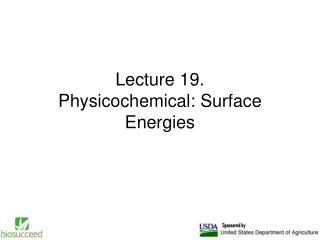DownloadDownload PresentationLecture 19. Physicochemical: Surface Energies

# Lecture 19. Physicochemical: Surface Energies

Télécharger la présentation## Lecture 19. Physicochemical: Surface Energies

- - - - - - - - - - - - - - - - - - - - - - - - - - - E N D - - - - - - - - - - - - - - - - - - - - - - - - - - -
##### Presentation Transcript

1. Lecture 19.Physicochemical: Surface Energies

2. Physicochemical: Surface Energies Surfaceenergy quantifies the disruption of intermolecular bonds that occurs when a surface is created. Surface energy may be defined as the excess energy at the surface of a material compared to the bulk.

3. Surface energy measurement of a liquid Surface energy is most commonly quantified using a contact angle goniometer and a number of different methods. Thomas Young described surface energy as the interaction between the forces of cohesion and the forces of adhesion which, in turn, dictate if wetting occurs. If wetting occurs, the drop will spread out flat. In most cases, however, the drop will bead to some extent and by measuring the contact angle formed where the drop makes contact with the solid the surface energies of the system can be measured.

4. Surface energy measurement of a liquid Young's equation

5. Surface energy measurement of a liquid

6. Surface energy measurement of a liquid γSL, γLV, and γSV are the interfacial tensions between the solid and the liquid, the liquid and the vapor, and the solid and the vapor, respectively.

7. Surface energy measurement of a liquid The Young equation assumes a perfectly flat surface, and in many cases surface roughness and impurities cause a deviation in the equilibrium contact angle from the contact angle predicted by Young's equation.

8. Surface energy measurement of a Solid The surface energy of a solid is usually measured at high temperatures. At such temperatures the solid creeps and even though the surface area changes, the volume remains approximately constant.

9. Surface energy measurement of a Solid If γ is the surface energy density of a cylindrical rod of radius r and length l at high temperature and a constant uniaxial tension P, then at equilibrium, the variation of the total Gibbs free energy vanishes and we have: G is the Gibbs free energy and A is the surface area of the rod

10. goniometer